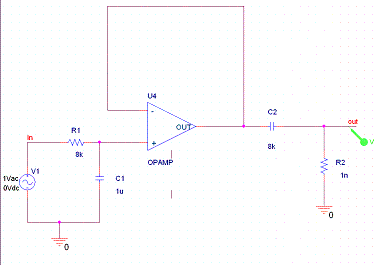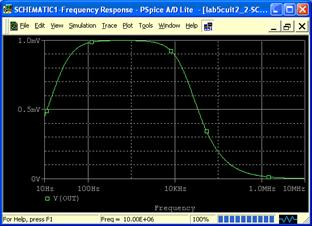Bill Chun Wai Hung

Q1)Find the values of R1, C1, R2, C2 Using the version of spice that you want, you should be able to describe the circuit and simulate it.

R1 = 8k ohm

R2 = 8k ohm

Low-Pass

f0 = 1/ (2*pi*R1C1) = 20Hz

High-Pass

f1 = 1/ (2*pi*R2C2) = 20kHz

Q2)Describe the circuit using Spice.with the source file

V_V1         IN 0 DC 0Vdc AC 1Vac

R_R2         0 OUT  1n

E_U4         N01107 0 VALUE {LIMIT(V(N00142,N01107)*1e13,-15V,+15V)}

R_R1         IN N00142  8k

C_C1         0 N00142  1u

C_C2         OUT N01107  8k

Q3)Set an AC analysis to verify the cutoff frequenciesThe AC Sweep shows a band-pass filter result with f0 = 20Hz and f1 =20kHz

The plot shows a shape of the bandpass filter result. In which the signal rises (20Hz) and drops later on (20kHz).

However, the Peak voltage in the plot is only 1.0mV. The cutoff voltage should be close to 0.7V instead of 1mV.